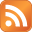# James Bond 007: Everything or Nothing PS2 cheatsCheat codes

Enter these codes at the pause menu you can get to while playing the game, after you have achieved the appropriate Platinum award.

Circle, Triangle, X, X, Circle - All Weapons
Circle, Triangle, X , Triangle, Square - Cloak
Circle, Circle, X, Circle, Triangle - Double ammunition
Circle, Triangle, Triangle, Square, Circle - Double damage
Circle, X, X, Square, Triangle - Easier movement in vehicle
Circle, Circle, Triangle, Square, Square - Full ammunition
Circle, Triangle, Triangle, X, Circle - Full battery
Circle, Triangle, X, Circle, Triangle - Golden Gun
Circle, Square, Square, X, Circle - Improved battery
Circle, Square, Square, Circle, X - Platinum Gun
Circle, Square, Triangle, X, Triangle - Slow motion driving
Circle, X, Square, X, Circle - Unlimited ammunition
Circle, Square, Circle, Square, Triangle - Unlimited Battery